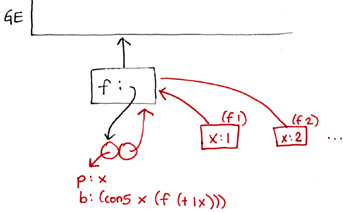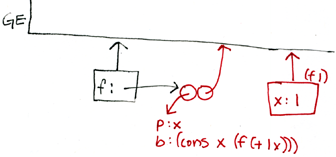```recitation 22 - lazy evaluation & streams
---------------------------------------------

(delay-it <exp> <env>) ;; Known as a 'thunk'

This is a promise to return a value when needed later or 'forced'.

(force-it <thunk>)

This forces the evaluation of a thunk or delayed expression.

Recall that it is possible to memoize a thunk by mutating it into an evaluated thunk.

* Why don't we always memoize thunks?

* Do applicative order and normal order evaluators always result in

----------------------------------------------------------

* What does the following expression evaluate to using applicative
order and normal order?

(letrec ((f (lambda (x) (cons x (f (+ 1 x))))))
(car (f 1)))

here's what the environment diagram looks like for applicative order:
``````  NOTE:  why do we have to use letrec?  if we instead tried to evaluate:

(let ((f (lambda (x) (cons x (f (+ 1 x))))))
(car (f 1)))

we'd get this diagram:
``````  and an error...  unbound variable f.

----------------------------------------------------------

* What does the following expression evaluate to using applicative order and
normal order?

(let* ((x 0)
(y 1)
(f1 (lambda () (begin (set! x (+ 1 x)) (* x y))))
(f2 (lambda () (begin (set! y (+ 1 y)) (* x y)))) )
(+ (f1) (f2)))

----------------------------------------------------------

Applicative Order - Call by Value (strict) < Scheme
------------------------------------------

- Simple and well understood.
- Predictable.
- Easily implemented.
- Interacts well with other languages.

- Doesn't allow infinite lists.
- May compute unneeded values.

Normal Order - Call by Need (lazy)
----------------------------------

- Infinite Lists.
- Never compute unneeded values.
- Don't get stuck in infinite recursions if return value not used.
- Used to easily implement if, and, or.

- Confusing to program.
- Difficult to predict, especially with side effects.
- Doesn't link well to other languages.

----------------------------------------------------------
Using Lazy Evaluation for streams:

Use Sequence manipulations without incurring the cost of manipulating sequences
of lists.  Works by only partially constructing streams, and then passing them
to a process that consumes the stream. If the consuming process requires more of
the stream that has not been evaluated, the stream automatically constructs just
enough more of itself to give the illusion that it completely exists.

(define (cons-stream x (y lazy-memo))
(cons x y))

(define stream-car car)

(define stream-cdr cdr)

--------------------------------------------------------------------

* What stream does the following expression return?

(define alt (cons-stream 0 (interleave integers alt)))

* Write a function that merges two ordered streams of integers, removing
duplicates as it goes. Assume that each individual stream contains no
duplicates.

(define (merge s1 s2)
<YOUR-CODE-HERE> )

--------------------------------------------------------------------

Recall the following procedure that returns a list of all the
distinct elements in a given list.  That is, each element
appears only once in the result list, no matter how many times it
appeared in the argument list.

(define (remove-duplicates lst)
(cond ((null? lst) '())
((null? (member (car lst) (cdr lst)))
(cons (car lst)
(remove-duplicates (cdr lst))))
(else (remove-duplicates (cdr lst)))))

* Write the analogous procedure that removes duplicates from a stream.

* Given a stream S and an element x, show how to use stream-filter to
remove all occurrences of x from S.

* Using the idea of above, write an alternative procedure to remove
duplicates from a stream.

--------------------------------------------------------------------

Signal Reconstruction and Phase Unwrapping
------------------------------------------

A phase wrapped signals are used in applications such as medical imaging and
radar.  Each signal value is measured modulus some range R. In this way, the
signal can be compressed or 'wrapped' into the range R. Phase unwrapping is used
to reconstruct the original measurement from this wrapped signal.

* Given a wrapped stream 'wrapped-str', write a proceedure to reconstruct the
original signal.

(define (phase-unwrap wrapped-str range)
<YOUR-CODE-HERE>)

* What assumptions must we make about the stream in-order for this to be
possible.
```